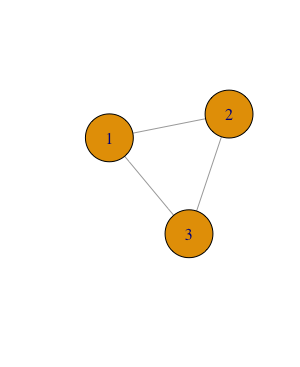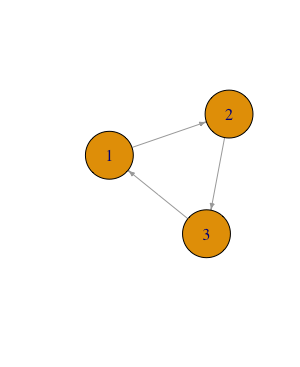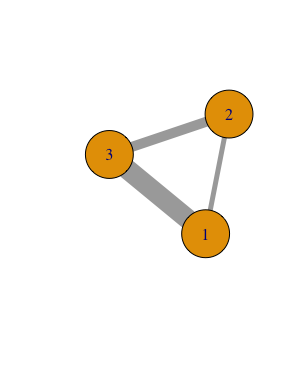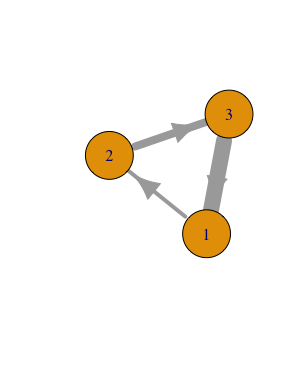## 5.2 Density and Other Structural Measures for Complete Networks

Carolan (2014), chapter 5 does a great job introducing a list of commonly used network-level measures.

Below, I make several extra points that are not covered:

First, the chapter is based on a `directed, non-weighted` network. We need to recognize that there are several types of networks based on the kinds of ties they have (see Table 5.19). Take the measure of `density` for example, the number of possible links are different for a directed network and an undirected network with the same `size`: a directed network has more possible links because each pair of nodes have two possible links. There are different algorithms (built in SNA tools) that would account for network types when calculating these measures.

Table 5.1: Different types of networks.
Undirected Directed
UnweightedWeightedSecond, the researcher sometimes need to make important decisions when computing network measures, even if a same algorithm is applied. For example, for a weighted network, it is a common practice to set a `threshold` of weight (based on some justified reasons) to filter links weaker than the threshold (see Example 4 from the previous page). Then network `density` could be computed the same way as an unweighted network. In this case, the calculated measure of density is highly sensible to the researcher’s decisions, which are influenced by her/his assumptions of the network. (Decision Point)

“It is important that a researcher does not use a measure simply because it is available in a standard program. A researcher must always be perfectly clear about the assumptions involved in any particular procedure, and must report these along with the density measures calculated” (Scott, 2013, p. 73)

As we’re diving into specific SNA techniques – such as `density`, `average degree` – I want to remind you of the “3 levels of considerations” we explored in Week 2. Decision points we’ve covered this week, such as which layout to use and what threshold to apply when computing density of a weighted network, are often informed by theoretical frameworks we adopt in a study or contextualized knowledge we have about a research problem. It is important for us to make our theoretical assumptions explicit in an SNA study.

1. See the R code I wrote to produce these toy networks.